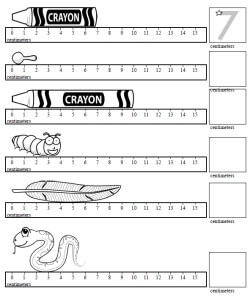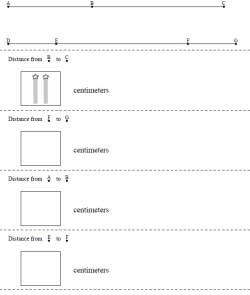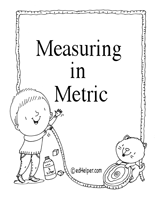Worksheets and No Prep Teaching Resources
Math Worksheets

# Metric System Worksheets

Understanding the metric system is an essential skill for students in today's connected world! These innovative worksheets cover the basics of the metric system in creative ways from word problems to crossword number puzzles and more! Vital concepts from length, weight, and capacity to conversions are covered in lively, exciting ways that will prepare students to go all around the world and to be prepared for any area of study in science or math that interests them!

Measuring LengthMeasure length in centimeters - first answer given

Measuring LengthMeasure length in centimeters

Length of Lines
CentimetersMeasure length in centimeters between points

Metric Problem Solving Puzzles
Temperatures
Kilometers per Liter
Measuring Volume
Measuring Volume (Has a Mix of Metric Measurements)
Calculating Density (No Decimals)
Calculating Density (Decimals)
Measuring Weight: Shipping Packages (Grams and Kilograms)

Metric Problem Word Stories
Metric System Word Stories Worksheet #1
Metric System Word Stories Worksheet #2
Metric System Word Stories Worksheet #3
Metric System Word Stories Worksheet #4
Metric System Word Stories Worksheet #5
Metric System Word Stories Worksheet #6
Metric System Word Stories Worksheet #7
Halloween Metric System Word Problems

Metric PizzaMeasuring in Metric

Metric System Worksheets
Metric Length
Metric Weight
Metric Capacity
Compare Metric Measurements
Mixed Metric System Measurement Conversions

Metric System Crossword Number Puzzles
Metric Length
Metric Weight
Metric Capacity
Mixed Metric Conversions
Convert Between Fahrenheit and Celsius
Mixed Metric Decimal Conversions

Have a suggestion or would like to leave feedback?